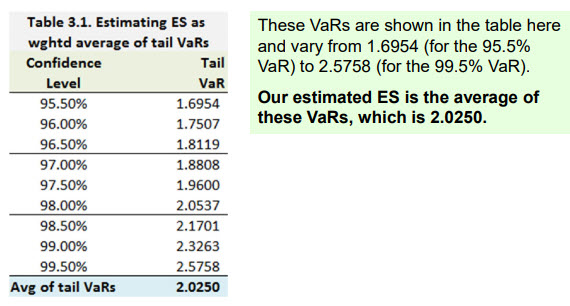# Calculation of Tail VaR in ES and Normal Deviate in Spectral Measure

#### Jul Jul

##### New Member
Subscriber
Hello,

I have just started with the first instructional video of part 2, covering market risk measures.

In the ES calculation, I do understand the concept, but what is confusing me after many hours of trying to figure it out, is the calculation of the tail VaRs. For example, for the 99% CL, the z-score of the normal distribution is (1.99/2)= 0.995. Using the z-score table, the normal deviate should be 2.575. Given that the mean is zero and the standard deviation is 1, normal VaR is -0 + 1*2.575 = 2.575. However, the corresponding tail VaR in the example is 2.3263.

The same applies for the normal deviate calculations, at different confidence levels, in the example of the estimation of exponential spectral risk measures as weighted average of VaRs. The corresponding normal deviates for each CL are nowhere near the true values. Please help, what can I be doing wrong?

Thanks.

#### David Harper CFA FRM

##### David Harper CFA FRM
Staff member
Subscriber
Hi @julienfrancaoui Please see below (I think this is the table/illustration to which you refer?). Please allow me to make two corrections to your example ...
For example, for the 99% CL, the z-score of the normal distribution is (1.99/2)= 0.995.
First, the confidence level for both VaR and expected shortfall (ES) is always one-tailed. So, a 99.00% CL refers to one-tail CL such that we do not "translate" it into 0.9950. The quantile associated with a 99.00% VaR is given by Excels' function =NORM.S.INV(0.990) = 2.3263. That is an inverse CDF: it accepts 99.0% as the probability and returns the associated cumulative quantile which is necessarily one-sided: the 1.0% tail is to the right of the 2.326 (i.e., one sided!). Second, the z-score is the quantile, not the probability; minor point. In this way, in terms of the standard normal distribution (by definition: zero mean and unit variance), at the quantile (aka, deviate) of +2.3263 it is the case the 99.0% of the distribution is to the left and 1.0% of the distributions area (under the pdf) is to the right, per the one-tailed perspective. I hope that's helpful, let me know if you require further clarification please?Last edited:

#### Jul Jul

##### New Member
Subscriber

Regarding the following statement: "The quantile associated with a 99.00% VaR is given by Excels' function =NORM.S.INV(0.990) = 2.3263"

Can you kindly explain how to obtain to the 2.3263 without using the excel function? I know this is something from part 1, but I am not recalling the method. My calculated 2.575 for the 99% CI is stated in several sources such as this one:

Of topic: I sent you message in private related to the accounting settings, and I would appreciate if you or your team can support in this inquiry.

#### Nicole Seaman

##### Director of FRM Operations
Staff member
Subscriber
Of topic: I sent you message in private related to the accounting settings, and I would appreciate if you or your team can support in this inquiry.

Hello @julienfrancaoui

Please make sure to send any emails to [email protected] or to me if you are sending a private forum message, as I am the person who works with all of the accounts and provides customer support for things like that. If you sent David a private message in the forum already, please add me to the conversation so I can help you. Thank you.

#### David Harper CFA FRM

##### David Harper CFA FRM
Staff member
Subscriber
Hi @julienfrancaoui Yea this is a basic skill that is already much-discussed in the forum; e.g., see https://forum.bionicturtle.com/threads/probability-function.23487/post-83885

See below. The Z-lookup table gives you the relationship between N(z) = p; for example (see red below) N(-2.33) = 0.0099 ≅1.0%. We need to be able to use this lookup table "interactively" by which I mean we need to be able to invert to get z = N^-1(p) as in -2.33 = N^-1(1.0%). Further, we need to be facile with the fact that all of these values are one-tailed CDFs, by definition. If we want a two-sided 99.0% confidence interval, then we want 2.58 at 99.0% (because if we want 1.0% to be shared between each tail, then it's the same as the one-tailed quantile at 0.50%, see purple below). VaR is always one-sided, but CIs are typically two-sided. Okay, I've now re-explained this for literally the one hundreth time on the forum! I hope it's helpful and feel free to use search in the future because it saves us all a lot of time when you look to see if a question has been previously asked/answered....Subscriber